# Fault-tolerant quantum computation with few qubits

## Abstract

Reliable qubits are difficult to engineer, but standard fault-tolerance schemes use seven or more physical qubits to encode each logical qubit, with still more qubits required for error correction. The large overhead makes it hard to experiment with fault-tolerance schemes with multiple encoded qubits. Here, we study the 15-qubit Hamming code, which protects seven encoded qubits to distance three. We give fault-tolerant procedures for applying arbitrary Clifford operations on these encoded qubits, using only two extra qubits, 17 in total. In particular, individual encoded qubits within the code block can be targeted. Fault-tolerant universal computation is possible with four extra qubits, 19 in total. The procedures could enable testing more sophisticated protected circuits in small-scale quantum devices. Our main technique is to use gadgets to protect gates against correlated faults. We also take advantage of special code symmetries, and use pieceable fault tolerance.

## Introduction

Quantum computers are faulty, but schemes to tolerate errors incur a large space overhead. For example, one qubit encodes into seven physical qubits using the Steane code,1 or into nine physical qubits using the Bacon-Shor and the smallest surface codes.2,3,4 Error correction uses additional qubits. When more than one level of encoding is required for better protection, the overhead multiplies, so that thousands of physical qubits can be required for each logical qubit.5 This overhead will compound the challenge of building large quantum computers. In the near term, it also makes it more difficult to run fault-tolerance experiments, which are important to test different schemes’ performance, validate models, and learn better approaches.

Codes storing multiple qubits have higher rates,6 but too large codes tend to tolerate less noise since initializing codewords gets difficult.7 A key obstacle for using any code with multiple qubits per code block is that it is complicated and inefficient to address the individual encoded qubits to compute on them.8,9 For example, to apply a CNOT gate between two logical qubits in a code block, the optimized method in Steane and Ibinson9 requires a full ancillary code block, with no stored data, into which the target logical qubit is transferred temporarily.

## Results

We introduce lower-overhead methods for computing fault tolerantly on multiple data qubits in codes of distance two or three.

1. 1.

For even n, the $$\left[\kern-0.15em\left[ {n,n - 2,2} \right]\kern-0.15em\right]$$ code encodes n − 2 logical qubits into n physical qubits, protected to distance two. We show that with two more qubits, encoded CNOT and Hadamard gates can be applied fault tolerantly. For n ≥ 6, four extra qubits suffice to fault-tolerantly apply an encoded CCZ gate, for universality.

2. 2.

For better, distance-three protection, we encode seven qubits into 15, and give fault-tolerant circuits for the encoded Clifford group using two more qubits, and for a universal gate set with four extra qubits (19 in total).

Combined with the two-qubit fault-tolerant error-detection and error-correction methods in Chao and Reichardt,10 this means that substantial quantum calculations can be implemented fault tolerantly in a quantum device with fewer than 20 physical qubits. Figure 1 summarizes our results.

In order to compute on data encoded within a single code block, we need to apply two- or three-qubit gates. The particular circuits use symmetries of the codes or a more general round-robin construction from Yoder et al.11 This is not fault tolerant, because a single-gate failure can cause a correlated error of weight two or worse, which a distance-three code cannot correct. To fix this, we replace each gate with a gadget involving two to four more qubits. With no gate faults, the gadgets are equivalent to the ideal gates they replace. The gadgets’ purpose is to detect correlated errors, so that they can be corrected for later; see Method for construction and properties of gadgets. The gadgets cannot prevent the gates from spreading single-qubit faults into problematic multi-qubit errors. To avoid this problem, we design the circuits carefully, and in some cases intersperse partial error-correction procedures between gadgets, an idea from Knill et al.12 recently applied and extended by Yoder and Hill et al.11,13 Sometimes error correction even needs to overlap the gadgets. See Discussion for details.

For the basics of stabilizer algebra, quantum error-correcting codes, and fault-tolerant quantum computation, we refer the reader to Gottesman.14

## Discussion

### Fault-tolerant operations for $$\left[\kern-0.15em\left[ {n,n - 2,2} \right]\kern-0.15em\right]$$ codes

For even n, the $$\left[\kern-0.15em\left[ {n,n - 2,2} \right]\kern-0.15em\right]$$ error-detecting code has stabilizers Xn and Zn, and logical operators $$\bar X_j$$ = X1Xj+1, $$\bar Z_j$$ = Zj+1Zn for j = 1, …, n − 2. This code, its symmetries, and methods of computing fault tolerantly on the encoded qubits were studied by Gottesman.8 However, his techniques require at least 2n extra qubits. For example, to apply a CNOT gate between two logical qubits in the same code block, he teleports them each into separate code block, applies transversal CNOT gates between the blocks, and then teleports them back.

We will give a fault-tolerant implementation of encoded CNOT and Hadamard gates on arbitrary logical qubits, using only two extra qubits. Two-qubit fault-tolerant procedures for state preparation, error detection, and projective measurement were given in Chao and Reichardt.10 For n ≥ 6 (so there are at least three encoded qubits), we will give a four-qubit fault-tolerant implementation of the encoded CCZ gate, thereby completing a universal gate set.

#### Permutation symmetries and transversal operations

Fault tolerance for a distance-two code means that any single fault within an operation should either have no effect or lead to a detectable error. For example, of course, the 4n−2 logical Pauli operators can all be applied fault tolerantly, since the operations do not couple any qubits.

All qubit permutations preserve the two stabilizers and therefore preserve the code space. They are also fault tolerant if implemented either by relabeling the qubits or by moving them past each other (and not by using two-qubit SWAP gates15). For i, j [n − 2], i ≠ j, the qubit swap (i + 1, j + 1) swaps the logical qubits i and j. The qubit swap (1, 2) implements logical CNOTs from qubits 2 through n − 2 into 1, and the qubit swap (2, n) implements logical CNOTs in the opposite direction: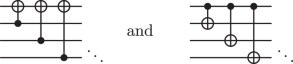Transversal Hadamard, Hn, followed by the qubit swap (1, n), implements logical H(n−2).

The Clifford reflection G = $${\textstyle{i \over {\sqrt 2 }}}(X + Y)$$ conjugates X ↔ Y and Z → −Z. Transversal G is a valid logical operation (up to Zn to correct the Xn syndrome if n = 2 mod 4). It implements logical CZ gates between all encoded qubits: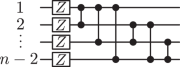The operations given so far generate a group much smaller than the full (n − 2)-qubit Clifford group. The qubit permutations generate n! different logical operations (except for n = 4, just 6 operations). With the transversal application of the six one-qubit Clifford gates, up to Paulis, this gives 6(n!) different logical operations (or 36 for n = 4). Table 1 gives the sizes of various interesting subgroups of the Clifford group, for comparison.

We next give fault-tolerant implementations for a logical Hadamard gate on a single encoded qubit, and for a logical CZ gate between two encoded qubits. These generate a large subgroup of the Clifford group, the $$\left\langle {{\mathrm{CNOT}},H} \right\rangle$$ column in Table 1.

#### CZ gate

By Claim 2, a logical CZ1,2 gate can be implemented by Zn CZ2,3 CZ2,n CZ3,n: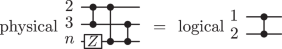(1)

However, this implementation is not fault tolerant. Some failures are detectable; for example, if the CZ2,3 gate fails as XI, then the final, detectable error is X2Zn. Others are not, e.g., if the CZ2,3 gate fails as XX, then the final X2X3 = $$\bar X_1\bar X_2$$ error is undetectable.

The bad faults that can cause undetectable logical errors are as follows:

CZ2,3 CZ2,n CZ3,n
Fault Error Fault Error Fault Error
ZZ Z 2 Z 3 ZZ Z 2 Z n ZZ Z 3 Z n
XX X 2 X 3 XY X 2 Z 3 Y n XX X 3 X n
YY Y 2 Y 3 YX Y 2 Z 3 X n YY Y 3 Y n

In particular, all these bad faults are caught by the CZ gadget of Theorem. Therefore, replacing each physical CZ gate in Eq. (1) with that gadget gives a fault-tolerant implementation of a logical CZ1,2 gate. The circuit uses at most two ancilla qubits at a time.

In fact, one can simplify the resulting circuit by using the same |0〉 ancilla to catch X faults on multiple CZ gates. The following circuit is also fault tolerant: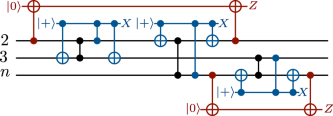The gadgets to catch Z faults can be merged, too. The following circuit is fault tolerant, and still requires at most two ancilla qubits at a time: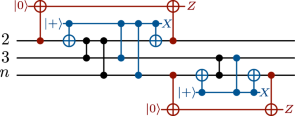(2)

Perhaps further simplifications are possible.

A single encoded Hadamard gate can also be implemented fault tolerantly with two extra qubits. The black portion of the circuit below, with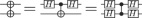, implements $$\bar H_1$$. The red and blue portions, analogous to Fig. 2a, b, respectively, catch problematic faults. X measurements should return + and Z measurements 0.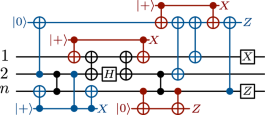(3)

The circuit’s fault tolerance can be verified by enumerating all the ways in which single gates can fail.

#### Four-ancilla CCZ gate

For n ≥ 6, a CCZ gate on encoded qubits 1, 2, 3 can be implemented by round-robin CCZ gates on {2, n} × {3, n} × {4, n}, by Claim 2: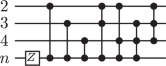(4)

This circuit uses one Z, three CZ, and four CCZ gates. To make it fault tolerant, use the gadget from Fig. 2c for each CZ gate, and replace each CCZ with the gadget of Eq. (15). Overall, this requires four ancilla qubits.

Single gate faults are either caught by the gadgets or lead to an error that could also arise from a one-qubit fault between the gates in Eq. (4). A one-qubit X or Y fault will be detectable at the end because it is copied only to linear combinations of Zs—the X component of the final error will still have weight one—and a one-qubit Z fault will be detectable because it commutes through the CZ and CCZ gates. Therefore the procedure is fault tolerant.

### Fault-tolerant operations for the $$\left[\kern-0.15em\left[ {15,7,3} \right]\kern-0.15em\right]$$ Hamming code

The $$\left[\kern-0.15em\left[ {15,7,3} \right]\kern-0.15em\right]$$ Hamming code is a self-dual CSS, perfect distance-three code. Packing seven logical qubits into 15 physical qubits, it is considerably more efficient than more commonly used $$\left[\kern-0.15em\left[ {7,1,3} \right]\kern-0.15em\right]$$ and $$\left[\kern-0.15em\left[ {9,1,3} \right]\kern-0.15em\right]$$ CSS codes, although it tolerates less noise.

We first give a presentation of the code and its symmetries following Harrington and Reichardt.16 Then, we give a two-ancilla-qubit method for fault-tolerantly implementing the full Clifford group on the encoded qubits, and, to complete a universal gate set, a four-qubit fault-tolerant encoded CCZ gate.

Two-qubit fault-tolerant procedures for state preparation and error correction were given in Chao and Reichardt.10

#### $$\left[\kern-0.15em\left[ {15,7,3} \right]\kern-0.15em\right]$$ Hamming code

The $$\left[\kern-0.15em\left[ {15,7,3} \right]\kern-0.15em\right]$$ Hamming code has four X and four Z stabilizers, each given by the following parity-checks:

$$\begin{array}{*{20}{c}} 0 & 0 & 0 & 0 & 0 & 0 & 0 & 1 & 1 & 1 & 1 & 1 & 1 & 1 & 1 \cr 0 & 0 & 0 & 1 & 1 & 1 & 1 & 0 & 0 & 0 & 0 & 1 & 1 & 1 & 1 \cr 0 & 1 & 1 & 0 & 0 & 1 & 1 & 0 & 0 & 1 & 1 & 0 & 0 & 1 & 1 \cr 1 & 0 & 1 & 0 & 1 & 0 & 1 & 0 & 1 & 0 & 1 & 0 & 1 & 0 & 1 \end{array}$$
(5)

Index the qubits left to right from 1 to 15. Observe that the columns are these numbers in binary.

As in Harrington and Reichardt,16 we define logical operators based on the following seven weight-five strings:

$$\begin{array}{*{20}{c}} 1 & 1 & 0 & 1 & 0 & 0 & 0 & 1 & 0 & 0 & 0 & 0 & 0 & 0 & 1 \cr 1 & 1 & 0 & 0 & 1 & 0 & 0 & 0 & 0 & 1 & 0 & 1 & 0 & 0 & 0 \cr 1 & 1 & 0 & 0 & 0 & 1 & 0 & 0 & 0 & 0 & 1 & 0 & 0 & 1 & 0 \cr 1 & 1 & 0 & 0 & 0 & 0 & 1 & 0 & 1 & 0 & 0 & 0 & 1 & 0 & 0 \cr 1 & 0 & 0 & 1 & 0 & 1 & 0 & 0 & 1 & 1 & 0 & 0 & 0 & 0 & 0 \cr 1 & 0 & 0 & 1 & 0 & 0 & 1 & 0 & 0 & 0 & 0 & 1 & 0 & 1 & 0 \cr 1 & 0 & 0 & 0 & 0 & 0 & 0 & 1 & 0 & 1 & 0 & 0 & 1 & 1 & 0 \end{array}$$
(6)

From the first string, $$\bar X_1$$ = XXIXIIIXIIIIIIX and $$\bar Z_1$$ = ZZIZIIIZIIIIIIZ. The remaining strings specify the logical operators $$\bar X_2$$, $$\bar Z_2$$ through $$\bar X_7$$, $$\bar Z_7$$. (Note that combinations of operators in Eq. (6), i.e., the last three, can have weight 3, which defines the code distance.)

#### Transversal operations

Transversal operations are automatically fault tolerant.

Transversal Pauli operators implement logical transversal Pauli operators. Indeed, transversal X, i.e., X15, preserves the code and implements transversal logical X, i.e., X7, on the code space, and similarly for Y and Z.

In fact, any one-qubit Clifford operator applied transversally preserves the code space and implements the same operator transversally on the encoded qubits. For example, since the logical operators are each self-dual, applying the Hadamard gates H15 implements logical H7.

Of course, since the code is CSS, transversal CNOT gates between two code blocks implement transversal logical CNOT gates on the code spaces. Furthermore, Paetznick and Reichardt17 shows that on three code blocks, transversal CCZ can be used to obtain a universal gate set. Here, however, we will consider only single code blocks and Clifford operations.

#### Permutation symmetries

Permutations of the qubits are also fault tolerant, either by physically moving the qubits or by relabeling them.

The code’s permutation automorphism group has order 20, 160, and is isomorphic to A8 and GL(4, 2).16,18 It is generated by the following three 15-qubit permutations:

$$\begin{array}{l}\sigma _1 = (1,2,3)(4,14,10)(5,12,9)(6,13,11)(7,15,8)\cr \sigma _2 = (1,10,5,2,12)(3,6,4,8,9)(7,14,13,11,15)\cr \sigma _3 = (1,10,15,3,8,13)(4,6)(5,12,11)(7,14,9)\end{array}$$
(7)

These permutations fix the code space, but act nontrivially within it. The permutations σ1 and σ2 apply the respective permutations (1, 2, 3) and (3, 4, 5, 6, 7) to the seven logical qubits. Together, these generate the alternating group A7 of even permutations.

The logical effect of σ3 is not a permutation. It is equivalent to the following circuit of 24 CNOT gates, in which gates with the same control wire are condensed:(8)

Thus, the first logical qubit is fixed, while for j {2, …, 7} and P {X, Y, Z}, Pj is mapped to $$\left( {\mathop {\prod}\nolimits_{j = 2}^7 {\kern 1pt} P_j} \right)P_{j + 1}$$, wrapping the indices cyclically. This is a six-qubit generalization of a four-qubit operator studied in Gottesman8 [Sec. 6]. (Like permutations, this operation has the property of being a valid transversal operation on any stabilizer code.)

#### CZ circuits based on permutation symmetries

Any permutation symmetry of the code can be turned into a CZ automorphism (Fig. 3a):

### Claim 1

For a self-dual CSS code, if σ is a qubit permutation that fixes the code space, then the circuit with a CZ gate from i to σ(i), for all iσ(i), fixes the code space up to Pauli Z corrections.

Proof. Z stabilizers commute with the CZ gates, so are preserved. An X stabilizer XS = $$\mathop {\prod}\nolimits_{i \in S} {\kern 1pt} X_i$$ is mapped to $$\mathop {\prod}\nolimits_{i \in S} \left( {X_iZ_{\sigma (i)}Z_{\sigma ^{ - 1}(i)}} \right)$$ = $$\pm X_SZ_{\sigma (S) \cup \sigma ^{ - 1}(S)}$$. Up to sign, this is a stabilizer, since $$Z_{\sigma (S)}Z_{\sigma ^{ - 1}(S)}$$ is a stabilizer.

For example, the physical circuit in Eq. (1) comes from the cyclic permutation (2, 3, n) of the $$\left[\kern-0.15em\left[ {n,n - 2,2} \right]\kern-0.15em\right]$$ code.

Applying Claim 1 to σ3 of Eq. (7), the two CZ gates for the cycle (4, 6) cancel out, leaving the gates (CZ1,10CZ10,15…CZ13,1)(CZ5,12CZ12,11CZ11,5)…. As shown in Fig. 3b, the effect is that of logical CZ gates following the cycle (2, 3, 4, 5, 6, 7).

Notice that the logical effect necessarily consists of encoded CZ gates, because logical Z operators are unchanged and logical X operators pick up Z components. Also, the map from Claim 1 is not a homomorphism from permutations into unitary circuits (as the circuits square to identity while the permutations may not).

### CZ gates {8, 9} to {10, 11} and {12, 13} to {14, 15}

The permutation (6, 7)(8, 10, 9, 11)(12, 14, 13, 15) fixes the code, and under Claim 1 corresponds to the eight CZ gates of Fig. 4a. Figure 4b gives their logical effect.

Using Magma,19 we compute that the group generated by this operation, the permutations σ1, σ2, σ3, and transversal H has the same size as the $$\left\langle {{\mathrm{CNOT}},H} \right\rangle$$ group on six qubits (about 1.001 · 1020). (Adding transversal G only triples the group size.) This hints that by working in a logical basis in which one qubit has $$\bar X$$ = X15 and $$\bar Z$$ = Z15 (operators fixed by the permutations and by Fig. 4a), perhaps arbitrary combinations of CNOT and H can be applied to the other six qubits. But no, only half the $$\left\langle {{\mathrm{CNOT}},H} \right\rangle$$ group can be reached.

The circuit of Fig. 4a is not clearly fault tolerant. (For example, an XX fault after the CZ9,11 gate gives the error X9X11, which is indistinguishable from X2.) We can use the gadgets from Fig. 2 for each CZ gate to obtain the circuit of Fig. 4c, shown with the trailing error correction. Two ancilla qubits are needed.

We claim that this compiled circuit is fault tolerant. This means that if the input lies in the code space, the compiled circuit has at most one fault (a two-qubit Pauli fault after a gate, or a one-qubit fault on a resting qubit), and the subsequent error correction is perfect; then the final outputs lie in the code space with no logical errors. To verify fault tolerance, there are two cases to check.

First, consider the case that, with at most one fault, all the gadget measurements give the trivial output (0 for a Z measurement, + for X). Since the gadgets catch two-qubit gate faults, we need to only check possible one-qubit faults between gates. Inequivalent fault locations are marked with stars in Fig. 4c. (Faults at other locations either cause the same errors, or will be caught.) In particular, entering error correction the possible error can be 1, X1, Z1, Y1, …, X15, Z15, Y15—from thelocations. Or, fromlocations, it can be XIZZ, YIZZ, IXZZ, IYZZ, ZZXI, ZZYI, ZZIX, ZZIY, and IZXI, IZYI, IZIX, IZIY fromlocations, on qubits 8, 9, 10, 11—and similarly for qubits 12 to 15. This gives 70 different errors total. All 70 have distinct syndromes, and therefore can be corrected. (This fact can be verified either by computing all the syndromes, or by observing from Eq. (5) that Z8Z9Z10Z11, Z12Z13Z14Z15, Z1Z6Z7, Z1Z8Z9, Z1Z12Z13, and Z1Z14Z15 are logical operators. Thus, for example, if you observe the Z syndrome 1000, for error X8, and the X syndrome 0001, for error Z1, you can safely correct X8Z10Z11. Z1X8 cannot occur.)

Note that the error-correction procedure needs to take into account the X and Z stabilizer syndromes together to decide what correction to apply. This can work because the $$\left[\kern-0.15em\left[ {15,7,3} \right]\kern-0.15em\right]$$ code is not a perfect stabilizer code: there are 28 possible syndromes but only 1 + 15 · 3 possible trivial or weight-one errors. (It is only perfect as a CSS code, i.e., the 24 Z stabilizer syndromes are exactly enough to correct the 1 + 15 possible trivial or weight-one X errors, and similarly for Z errors.) This leaves room to correct some errors of weight more than one.

Next, consider the case that, with at most one fault in Fig. 4c, one or more of the gadget measurements gives a nontrivial output. This case is much simpler, because the measurement results localize the fault, leaving only a few possibilities for the error entering error correction. One must verify that in all cases, these possibilities are distinguished by their syndromes.

For example, if the first Z measurement returns 1 and all other measurements are trivial, the errors from single faults that can occur are, on qubits 8, 9, 10, 11:

$$\begin{array}{*{20}{r}} \hfill {IIII,} & \hfill {ZIII,} & \hfill {XIII,} & \hfill {YIII,}\cr \hfill {XIIZ,} & \hfill {YIIZ,} & \hfill {XIZZ,} & \hfill {YIZZ,}\cr \hfill {XZIX,} & \hfill {XZIY,} & \hfill {XZXZ,} & \hfill {XZYZ,}\cr \hfill {YZIX,} & \hfill {YZIY,} & \hfill {YZXZ,} & \hfill {YZYZ}\end{array}$$

These 16 possible errors all have distinct syndromes, so they are correctable.

As another example, if the last two measurements, of qubits coupled to qubit 13, are nontrivial, then the possible errors from single faults are, on qubits 12, 13, 14, 15:

$$IXII,IYII,IXIZ,IYIZ,IXIX,IXIY$$

Again, these have distinct syndromes.

Other possible measurement outcomes are similar. We have used a computer to check them all.

#### Round-robin CZ circuits to complete the Clifford group

The above operations do not generate the full seven-qubit logical Clifford group, and we have not been able to find a permutation for which applying Claim 1 enlarges any further the generated logical group. Instead, we turn to the round-robin construction of Claim 2.

### CZ gates 4 to {5, 6, 7}, 8 to {9, 10, 11}, and 12 to {13, 14, 15}

Observe that Z{4,8,12} and Z{4,5,6,7} ~ Z{8,9,10,11} ~ Z{12,13,14,15} are logical operators, implementing respectively $$\bar Z$${2,5,7} and $$\bar Z$${1,2,3,4}. By a minor extension of Claim 2, applying Z{4,8,12} and nine CZ gates from 4 to each of qubits {5, 6, 7}, 8 to {9, 10, 11}, and 12 to {13, 14, 15} preserves the code space. The logical effect is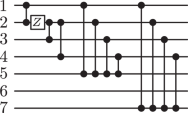(9)

Together with permutations and transversal operations, this circuit completes the seven-qubit Clifford group, without needing the operation from Fig. 4. To make the operation fault tolerant, we will transform it in three steps.

First, consider the circuit below, in which we have wrapped the CZ gates leaving qubits 4, 8, and 12 with overlapping gadgets to catch X faults. If at most one fault occurs and one or more of the Z measurements gives 1, then the errors that can occur are distinguished by their syndromes.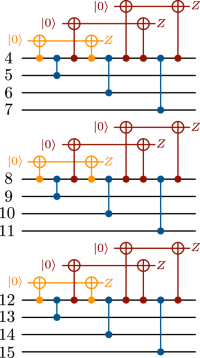(10)

For example, if the orange, first Z measurement off qubit 4 gives 1 and all others 0, then the possible errors entering error correction are, on qubits 4, 5, 6, 7:

$$\begin{array}{*{20}{r}} \hfill {IIII,} & \hfill {ZIII,} & \hfill {} & \hfill {}\cr \hfill {XIZZ,} & \hfill {XXZZ,} & \hfill {XYZZ,} & \hfill {XZZZ,}\cr \hfill {YIZZ,} & \hfill {YXZZ,} & \hfill {YYZZ,} & \hfill {YZZZ}\end{array}$$

All these errors have distinct syndromes.

The circuit in Eq. (10) is not fault tolerant, however, because with at most one fault if all the Z measurements give 0, some inequivalent errors will have the same syndrome. We can list the problematic errors. For each of the following sets, the errors within the set are all possible, but have the same syndrome:

$$\begin{array}{*{20}{c}} {\left\{ {Z_1,Z_4Z_5,Z_8Z_9,Z_{12}Z_{13}} \right\}} \cr {\left\{ {Z_2,Z_4Z_6,Z_8Z_{10},Z_{13}Z_{14}} \right\}} \cr {\left\{ {Z_3,Z_4Z_7,Z_8Z_{11},Z_{12}Z_{15}} \right\}} \cr {\left\{ {X_4,Y_4Z_5Z_6Z_7} \right\},\left\{ {Y_4,X_4Z_5Z_6Z_7} \right\}} \cr {\left\{ {X_8,Y_8Z_9Z_{10}Z_{11}} \right\},\left\{ {Y_8,X_8Z_9Z_{10}Z_{11}} \right\}} \cr {\left\{ {X_{12},Y_{12}Z_{13}Z_{14}Z_{15}} \right\},\left\{ {Y_{12},X_{12}Z_{13}Z_{14}Z_{15}} \right\}} \end{array}$$
(11)

Next, replace each of the blue CZ gates in Eq. (10) with the ZZ fault gadget from Fig. 2b. This gadget has the property that, with at most one failure, a ZZ fault can only occur if the X measurement returns −. These measurements thus distinguish the errors in the first three sets above.

Yet the new circuit is still not fault tolerant. The gadget measurements cannot distinguish the errors in each of the last six sets in Eq. (11). For example, consider an X4 error before the circuit. It propagates to X4Z5Z6Z7. Since Z4Z5Z6Z7 is a logical error, X4Z5Z6Z7 is indistinguishable from a Y4 error after the circuit, and no error-correction rules can correct for the possible logical error.

Gadgets cannot protect against single-qubit faults that occur just before or after the circuit. This circuit is qualitatively different from the one we considered in Fig. 4, and a new trick is needed to make it fault tolerant.

Consider the following circuit from Chao and Reichardt,10 ignoring for now the orange portion at the top right: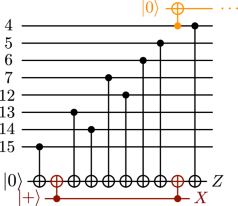(12)

This circuit fault-tolerantly extracts the syndrome of the Z{4,5,6,7,12,13,14,15} stabilizer, in the sense that

• With no gate faults, the Z measurement gives the syndrome, and the X measurement gives +.

• With at most one fault, if the X measurement gives +, then the data error has weight ≤1.

• With at most one fault, if the X measurement gives −, then the X component of the data error has weight ≤1. The Z component can be any of

$$\begin{array}{*{20}{c}} {Z_4,Z_{\{ 4,5\} },Z_{\{ 4,5,6\} },Z_{\{ 4,5,6,12\} },Z_{\{ 4,5,6,7,12\} }\sim Z_{\{ 13,14,15\} },} \cr {Z_{\{ 4,5,6,7,12,14\} }\sim Z_{\{ 13,15\} },Z_{\{ 4,5,6,7,12,13,14\} }\sim Z_{15},{\mathbf{1}}} \end{array}$$

and all these errors have distinct syndromes. (The order of the CNOT gates ensures this property.)

In Chao and Reichardt,10 this circuit was used in a two-ancilla-qubit fault-tolerant error-correction procedure.

The circuit in Eq. (12) is useful for us now to detect an X4 or Y4 error on the input to Eq. (10). However, it is not enough to measure the Z syndrome, or even to run the full error correction, before applying the circuit (10), because an X4 or Y4 fault could happen after the syndrome measurement completes and before Eq. (10). This problem is solved by the orange portion of Eq. (12), which is meant to continue into Eq. (10), replacing the first $$\left| 0 \right\rangle$$ preparation and CNOT. It gives qubit 4 temporary protection, so that an X4 or Y4 fault is caught by either the syndrome measurement or the orange Z measurement, or both.

While the above arguments give intuition for the construction, they leave out the details. Let us now present the full fault-tolerant construction.

1. 1.

Start by applying Eq. (12) to extract the syndrome for Z{4,5,6,7,12,13,14,15}. If the Z or X measurement is nontrivial, then decouple the orange qubit with another CNOT, apply error correction, and finish by applying unprotected CZ gates 4 to {5, 6, 7}, 8 to {9, 10, 11}, and 12 to {13, 14, 15}. (This is safe because one fault has already been detected.)

2. 2.

Next, if the Z and X measurements were trivial, apply the top third of circuit (10), where the orange qubit wire continues from Eq. (12), to implement protected CZ gates 4 to {5, 6, 7}. If any measurements are nontrivial, then finish by applying unprotected CZ gates 8 to {9, 10, 11} and 12 to {13, 14, 15}, then error correction. We have already argued that this is fault tolerant; the extended orange “flag” is enough to catch X4 or Y4 faults between Eqs. (12) and (10).

3. 3.

If the measurements so far were trivial, then apply a circuit analogous to Eq. (12) to extract the Z{8,9,10,11,12,13,14,15} syndrome. (Note that this is still a stabilizer, even though the CZ gates 4 to {5, 6, 7} have changed the code.) If the Z syndrome or X measurement is nontrivial, then apply error correction—a simple error-correction procedure is to apply CZ gates 4 to {5, 6, 7} to move back to the $$\left[\kern-0.15em\left[ {15,7,3} \right]\kern-0.15em\right]$$ code and correct there—before finishing with CZ gates 8 to {9, 10, 11} and 12 to {13, 14, 15}. If the Z and X measurements were trivial, then apply the middle portion of Eq. (10), where the orange qubit wire extends from qubit 8, to implement protected CZ gates 8 to {9, 10, 11}. If any measurements are nontrivial, then finish by applying unprotected CZ gates from 12 to {13, 14, 15}, and then error correction.

4. 4.

If the measurements so far were trivial, then extract the Z{8,9,10,11,12,13,14,15} syndrome using Eq. (12) except with the data qubits in order 12, 13, 14, 15, 8, 9, 10, 11 top to bottom (so that the orange flag attaches to qubit 12). If the Z or X measurement is nontrivial, then with at most one fault whatever error there is on the data can be corrected. (The easiest way is to move forward to the $$\left[\kern-0.15em\left[ {15,7,3} \right]\kern-0.15em\right]$$ code using CZ gates 12 to {13, 14, 15} and correct there. Note that these CZ gates turn the weight-one errors X12, X13, X14, X15 into X12Z{13,14,15}, Z12X13, Z12X14, Z12X15, respectively, but these can still be corrected; e.g., if in X error correction you detect X12, apply the correction X12Z{13,14,15}.) If the Z and X measurements were trivial, then apply the bottom portion of Eq. (10) to implement protected CZ gates from 12 to {13, 14, 15}, and correct the errors based on the measurement results.

Observe that this procedure requires two ancilla qubits.

#### Four-qubit fault-tolerant CCZ for universality

In order to realize a universal set of operations on the seven encoded qubits, we give a four-ancilla-qubit fault-tolerant implementation for an encoded CCZ gate. The idea is to start with a circuit of round-robin CCZ gates to implement the encoded CCZ non-fault-tolerantly (Claim 2), and then replace each CCZ with the gadget of Eq. (15) to catch correlated errors. Finally, we intersperse X error-correction procedures to catch X faults before they can spread, much like the pieceable fault-tolerance constructions of Yoder et al.11 except on a single code block. (A similar approach can also be used to implement encoded CZ gates.)

By Claim 2, an encoded CCZ gate can be implemented by round-robin CCZ gates on qubits {1, 4, 5} × {1, 6, 7} × {1, 8, 9} (one Z, six CZ, and 21 CCZ gates):(13)

To make this circuit fault tolerant, first replace each CZ gate with the gadget from Fig. 2c, and replace each CCZ gate with the gadget from Eq. (15). After each gadget, apply X error correction, and at the end apply both X and Z error correction. (As in Yoder et al.,11 it might be possible to put multiple CCZ gadgets before each X error correction, but we have not tried to optimize this.) Observe that X error correction can be implemented even partially through the round-robin circuit because the code’s Z stabilizers are preserved by CCZ gates.

There are two cases to consider to demonstrate fault tolerance: either a gadget is “triggered” with a nontrivial, 1 or −, measurement outcome, or no gadgets are triggered.

1. 1.

A gadget is triggered. If a gadget is triggered, then any Pauli errors can be present on its output data qubits. It is straightforward to check mechanically that for each CZ gate in Eq. (13), all four possible X errors, II, IX, XI, and XX, have distinct Z syndromes, and so can be corrected immediately in the subsequent X error correction, before the errors can spread. By symmetry, the four possible Z errors have distinct syndromes. These errors commute through Eq. (13) and are fixed by the final Z error correction.

Similar considerations hold for each CCZ gate: the possible X and Z error components have distinct syndromes, so an error’s X component can be corrected immediately and the Z component corrected at the end.

2. 2.

No gadgets are triggered. If there is a single failure in a CZ or CCZ gadget, but the gadget is not triggered, then the error leaving the gadget is a linear combination of the same Paulis that could result from a one-qubit X, Y, or Z fault before or after the gadget.

If the error has no X component, then as a weight-one Z error it commutes to the end of Eq. (13), at which point Z error correction fixes it.

If the error has X component of weight one, then the Z component can be a permutation of any of III, IIZ, IZZ, ZZZ on the three involved qubits (or of II, IZ, ZZ for a CZ gadget). As we have already argued, these Z errors have distinct X syndromes. The X error correction immediately following the gadget will catch and correct the error’s X component, keeping it from spreading. The final Z error correction, alerted to the X failure, will correct the error’s Z component.

### Remarks

Space-saving techniques for fault-tolerant quantum computation should be useful both for large-scale quantum computers and for nearer-term fault-tolerance experiments. Our techniques can likely be optimized further, and adapted to experimental model systems—but it might also be useful to relax the space optimization and allow a few more qubits, especially when memory error rates are comparable to those of physical gates and thus parallelization is desirable.

The techniques can also likely be applied to other codes, especially distance-three CSS codes. The round-robin CZ and CCZ constructions apply to some non-CSS codes, such as the $$\left[\kern-0.15em\left[ {8,3,3} \right]\kern-0.15em\right]$$ code, but then they are more difficult to make fault tolerant. It is an interesting open problem to devise fault-tolerant flag gadgets for unitary gates (rather than CZ or CCZ), whose universality is more efficient than that of orthogonal gates.

## Methods

A main trick we use is to replace CZ and CCZ gates with small gadgets that can catch correlated faults. The gadgets are reminiscent of one-ancilla-qubit fault-tolerant SWAP gate gadgets.15

The controlled-phase gate is a two-qubit diagonal gate CZ = 1 – 2$$\left| {11} \right\rangle \left\langle {11} \right|$$, represented in circuits asObserve that CZ gates commute with Z errors, but copy X (or Y) errors on one wire into Z errors on the other: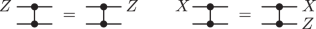(14)

For fault tolerance, it suffices to study gates that fail with Pauli faults after the gate. That is, when a noisy CZ gate fails, it applies the ideal CZ gate followed by one of the 15 nontrivial two-qubit Pauli operators.

Consider the circuit of Fig. 2a, using one extra qubit that at the end is measured in the $$\left| 0 \right\rangle ,\left| 1 \right\rangle$$ basis (Z eigenbasis). If the gates are perfect, then the measurement returns 0 and this circuit has the effect of a single CZ gate. If the CZ gate fails with an X or Y fault on the second qubit, however, then the measurement will return 1. Thus, certain kinds of faults can be detected. If at most one location fails and the measurement returns 0, then the output cannot have an XX, XY, YX, or YY error.

A similar circuit can catch Z faults. If all gates in Fig. 2b are perfect, then the X basis ($$\left| + \right\rangle ,\left| - \right\rangle$$) measurement will return + and the effect will be of a single CZ. If there is at most one fault and the measurement returns +, then the output cannot have a YX or ZZ error. (This fact can be verified by, e.g., propagating ZZI and ZZX backward through the circuit, and observing that no single gate failure can create either.)

The gadgets to catch X and Z faults can be combined:

#### Theorem

With no faults, the circuit of Fig. 2c implements a CZ gate, with the measurements outputting 0 and +. If there is at most one fault and the measurements return 0 and +, then neither XX, XY, YX, YY nor ZZ errors can occur on the output.

Thus, all single faults are caught except those equivalent to a fault on the CZ output or input qubits (namely, IX, IY, IZ, XI, YI, ZI and XZ, YZ, ZX, ZY). No CZ gadget can catch more errors than this.

The order of the gates matters. Although the last two gates in Fig. 2b formally commute, switching them changes the faulty circuit so that an undetected ZZ error can occur due to a single gate fault. Similarly, in Fig. 2c, it is important that the gadget for catching Z faults goes inside that for X faults. With the other order, a single fault can lead to an undetected XX error. See Fig. 5.

In practice, not every CZ gate need always be replaced with the full CZ gate gadget of Fig. 2c. Multiple gates can sometimes be combined under single flags. We will see examples below.

The three-qubit gate CCZ = 1 − 2$$\left| {111} \right\rangle \left\langle {111} \right|$$ is denoted asAgain, it commutes with Z errors. It copies an X error on the input into a CZ = $${\textstyle{1 \over 2}}(II + IZ + ZI - ZZ)$$ error on the output, i.e., into a linear combination of II, IZ, ZI and ZZ errors:The following gadget, using four ancilla qubits, implements a CCZ on the black data qubits. Furthermore, it satisfies that provided there is at most one failed gate and the measurement results are trivial, 0 and +, then the error on the output is a linear combination of Paulis that could result from a one-qubit fault before or after a perfect CCZ gate, i.e., III, ZII, XII, YII, XZI, YZI, XZZ, YZZ and qubit permutations thereof.(15)

That without faults the black and blue gates that realize a CCZ is a special case of the following claim,11 with r = 3, $$S_1^Z = \left\{ 1 \right\}$$, $$S_2^Z = \left\{ {2,3} \right\}$$, $$S_3^Z = \left\{ {5,6} \right\}$$.

#### Claim 2

Consider an n-qubit CSS code with k encoded qubits given by $$\bar X_j = X_{S_j^X}$$, $$\bar Z_j = Z_{S_j^Z}$$ for $$S_j^X,S_j^Z \subseteq [n]$$. Let U be the product of C(r−1)Z = 1 2$$\left| {1^r} \right\rangle \left\langle {1^r} \right|$$ gates applied to every tuple of qubits in $$S_1^Z \times S_2^Z \times \cdots \times S_r^Z$$. (If the $$S_j^Z$$ sets are not disjoint, then some of the applied gates will be C(s−1)Z for some s<r.)

Then U is a valid logical operation. It implements logical C(r−1)Z on the first r encoded qubits.

Proof. For the case that the sets $$S_j^Z$$ are disjoint, the claim is immediate in the case of singleton sets and follows in general by induction on the set size using the identity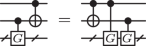for any gate G, on one or more qubits, with G2 = 1. (Fig. 2b provides one example.)

More generally, the claim follows by writing out computational basis codewords as superpositions of computational basis states, and computing the effect of U. For (x, y)  {0, 1}r × {0, 1}kr, the codeword $$\left| {\overline {xy} } \right\rangle$$ is a uniform superposition of computational basis states:

$$\left| {\overline {xy} } \right\rangle \propto \mathop {\prod}\limits_{j = 1}^r {\kern 1pt} {\overline{X}}_j^{x_j}\mathop {\prod}\limits_{i = r + 1}^k {\kern 1pt} {\overline{X}}_i^{y_{i - r}}\mathop {\sum}\limits_{{\mathrm{stabilizers}}{\kern 1pt} {\mathrm{X}}_{\mathrm{S}}} {\kern 1pt} X_S\left| {0^n} \right\rangle$$

The relationships $$\bar X_i\bar Z_j$$ = $$( - 1)^{\delta _{ij}}\bar Z_j\bar X_i$$ imply that $$\left| {S_i^X \cap S_j^Z} \right|$$ is odd for i = j and even otherwise; and for any stabilizer XS, [XS, $$\bar Z_j$$] = 0 so $$\left| {S \cap S_j^Z} \right|$$ is even.

If x ≠ 1r, say xj = 0, then for every term $$\left| z \right\rangle$$ in the above sum, z has an even number of 1s in $$S_j^Z$$, implying that $$U\left| z \right\rangle = \left| z \right\rangle$$. Hence $$U\left| {\overline {xy} } \right\rangle = \left| {\overline {xy} } \right\rangle$$.

If x = 1r, then for any term $$\left| z \right\rangle$$ in $$\left| {\overline {xy} } \right\rangle$$, z has an odd number of 1s in each $$S_j^Z$$ and therefore $$U\left| z \right\rangle = - \left| z \right\rangle$$. Hence $$U\left| {\overline {xy} } \right\rangle = - \left| {\overline {xy} } \right\rangle$$.

## Data availability

Data sharing is not applicable to this article as no datasets were generated or analyzed during the current study.

## References

1. 1.

Steane, A. M. Error correcting codes in quantum theory. Phys. Rev. Lett. 77, 793–797 (1996).

2. 2.

Bacon, D. Operator quantum error-correcting subsystems for self-correcting quantum memories. Phys. Rev. A. 73, 012340 (2006).

3. 3.

Bombn, H. & Martin-Delgado, M. A. Optimal resources for topological two-dimensional stabilizer codes: Comparative study. Phys. Rev. A. 76, 012305 (2007).

4. 4.

Horsman, C., Fowler, A. G., Devitt, S. & Van Meter, R. Surface code quantum computing by lattice surgery. New J. Phys. 14, 123011 (2012).

5. 5.

Suchara, M. et al. Comparing the overhead of topological and concatenated quantum error correction, Proc. IEEE Int. Conf. Comput. Design, https://doi.org/10.1109/ICCD.2013.6657074 (2013).

6. 6.

Calderbank, A. R. & Shor, P. W. Good quantum error-correcting codes exist. Phys. Rev. A. 54, 1098–1106 (1996).

7. 7.

Steane, A. M. Overhead and noise threshold of fault-tolerant quantum error correction. Phys. Rev. A. 68, 042322 (2003).

8. 8.

Gottesman, D. Theory of fault-tolerant quantum computation. Phys. Rev. A. 57, 127 (1998).

9. 9.

Steane, A. M. & Ibinson, B. Fault-tolerant logical gate networks for Calderbank-Shor-Steane codes. Phys. Rev. A. 72, 052335 (2005).

10. 10.

Chao, R. & Reichardt, B. W. Error correction with only two extra qubits. Phys. Rev. Lett 121, 050502 (2018).

11. 11.

Yoder, T. J., Takagi, R. & Chuang, I. L. Universal fault-tolerant gates on concatenated stabilizer codes. Phys. Rev. X 6, 031039 (2016).

12. 12.

Knill, E., Laflamme, R. & Zurek, W. H. Threshold accuracy for quantum computation, http://xxx.lanl.gov/abs/quant-ph/9610011 (1996).

13. 13.

Hill, C. D., Fowler, A. G., Wang, D. S. & Hollenberg, L. C. L. Fault-tolerant quantum error correction code conversion. Quant. Inf. Comput. 13, 439–451 (2013).

14. 14.

Gottesman, D. An introduction to quantum error correction and fault-tolerant quantum computation. Quantum Information Science and Its Contributions to Mathematics, Proc. Symp. in Applied Mathematics 68, (13–58. Amer. Math. Soc., Providence, RI, 2010).

15. 15.

Gottesman, D. Fault-tolerant quantum computation with local gates. J. Mod. Opt. 47, 333–345 (2000).

16. 16.

Harrington, J. & Reichardt, B. W. Addressable multi-qubit logic via permutations. Talk at Southwest Quantum Information and Technology (SQuInT) (2011)

17. 17.

Paetznick, A. & Reichardt, B.W. Universal fault-tolerant quantum computation with only transversal gates and error correction. Phys. Rev. Lett. 111, 090505 (2013).

18. 18.

Grassl, M. & Roetteler, M. Leveraging automorphisms of quantum codes for fault-tolerant quantum computation. In Proc. IEEE Int. Symp. on Information Theory, 534–538 (2013). http://arxiv.org/abs/arXiv:1302.1035.

19. 19.

Bosma, W., Cannon, J. & Playoust, C. The Magma algebra system I: The user language. J. Symb. Comput. 24, 235–265 (1997).

## Acknowledgements

We thank Ted Yoder for helpful comments. The research was supported by NSF grant CCF-1254119 and ARO grant W911NF-12-1-0541.

## Author information

Authors

### Contributions

All authors researched, collated, and wrote this paper.

### Corresponding author

Correspondence to Rui Chao.

## Ethics declarations

### Competing interests

The authors declare no competing interests.

Publisher's note: Springer Nature remains neutral with regard to jurisdictional claims in published maps and institutional affiliations.

## Rights and permissions

Reprints and Permissions

• ### Error detection on quantum computers improving the accuracy of chemical calculations

• Miroslav Urbanek
• , Benjamin Nachman
•  & Wibe A. de Jong

Physical Review A (2020)

• ### Error-transparent operations on a logical qubit protected by quantum error correction

• Y. Ma
• , Y. Xu
• , X. Mu
• , W. Cai
• , L. Hu
• , W. Wang
• , X. Pan
• , H. Wang
• , Y. P. Song
• , C.-L. Zou
•  & L. Sun

Nature Physics (2020)

• ### Pieceable fault tolerant conversion between 5-qubit code and 7-CSS code

• Chen Lin
• , GuoWu Yang
• , QingBin Luo
•  & XiaoYu Li

Quantum Information Processing (2020)

• ### Triangular color codes on trivalent graphs with flag qubits

• Christopher Chamberland
• , Aleksander Kubica
• , Theodore J Yoder
•  & Guanyu Zhu

New Journal of Physics (2020)

• ### Quantum information processing and quantum optics with circuit quantum electrodynamics

• Alexandre Blais
• , Steven M. Girvin
•  & William D. Oliver

Nature Physics (2020)

## Search